## Screenshots

•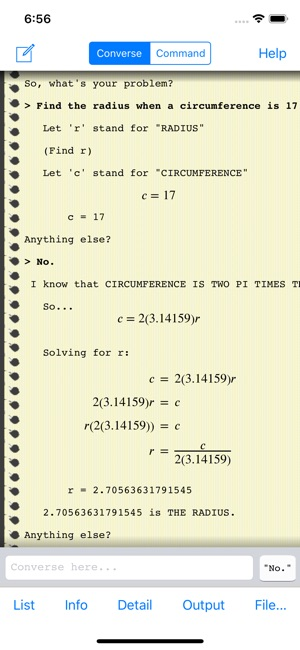•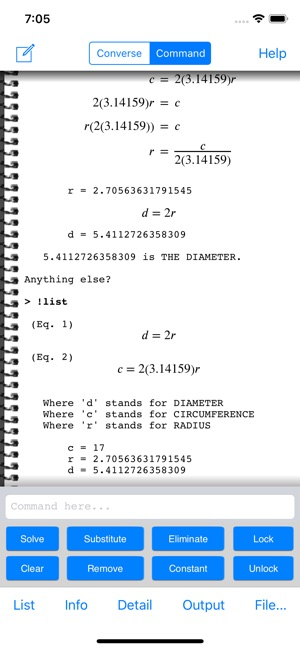•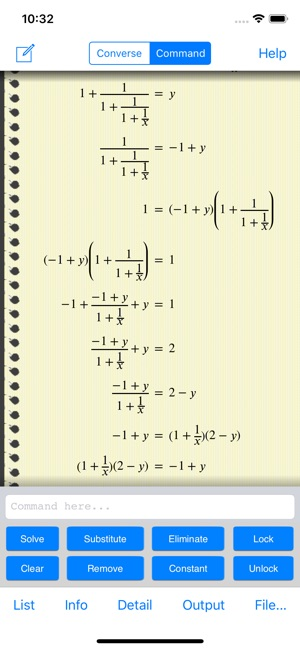•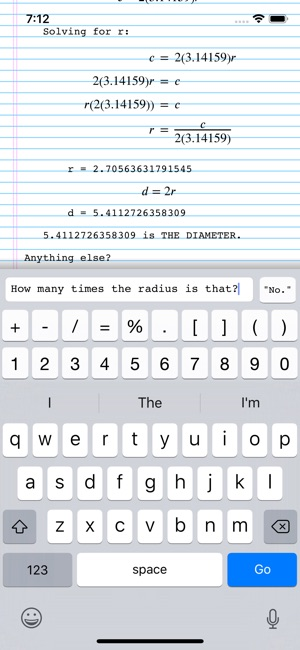•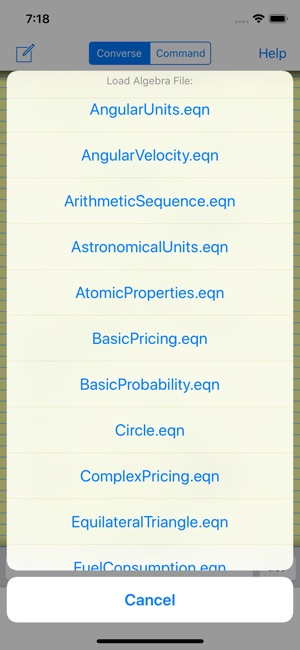•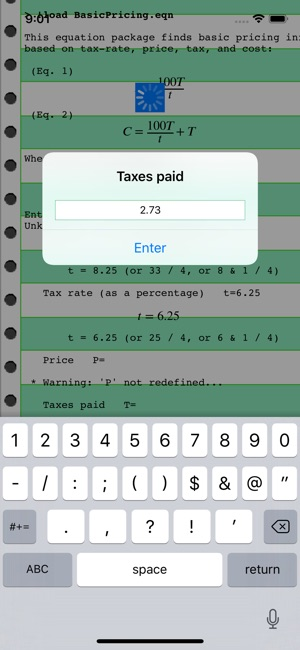## Description

AutoMathic is a different kind of calculator, smart enough to help you solve math problems by automating as much of the work as you want. With AutoMathic, you focus on specifying the problem, and let the computer do the mechanical work of solving it! If your current calculator can't directly solve for input like this, you need AutoMathic:

"How many millions is 12% of 275 million?"
"What must the price have been assuming that tips were 2.75?"
"21 is the difference between what circumference and what radius?"
"How many times 2.4[mHz] is 3.1[gHz]?"
"Find the cost daily when the cost semiannually is 475."
"3[drops/sec] converts to how many [gallons/day]?"
"If the average speed is 50[mph], and the radius is 50[m], how many G's would the centripetal acceleration be?"
"If the wave speed is the speed of sound, and the frequency is F#, how many unit inches long is the wavelength?"
"How many trillionths of a unit Coulomb is the charge when the capacitance is 53[pF], and the voltage is 12[V]?"
"If the power is 40[W], and the voltage is 12[V], find the resistance measured in ohms, and the current measured in amps."
"Find the mass per particle measured in AMU when the mass for 6 moles is 84[gm]."
"If the formula weight is 106, find the number of grams such that the molar concentration is 4 when the number of ml is 1,500."
"If the density is half the density of steel, and 75% of the volume is 2[gal], find the mass measured in pounds."
"If the specific gravity is 0.668, find the density measured in lb/gal."
"Find the number of nucleons, and the atomic weight. The atomic number is 6, the ratio of protons to neutrons is 1:1, and the ionic charge is +2."
"Compute the volume measured in liters if the number of moles is 1, pressure is standard pressure, and temperature is standard temperature."
"Find the heat energy measured in Joules given that the mass is 7.05[gm], deltaT is 75, and the specific heat is the specific heat of iron."

AutoMathic is great for:
- Math and Science Students
- Teachers and Tutors
- Scientists and Engineers
- "Everyday" math
- "Off-the-cuff" calculations
- Anyone who's naturally curious or likes to play with numbers!

Calculate *your* way, with 3 complementary modes of operation:

1. AutoMathic lets you run scripted calculations from a large, built-in library if you want to do something commonplace.

2. AutoMathic lets you do manual calculations by accepting expressions and equations you enter yourself, and gives you the tools to manipulate them using familiar techniques from algebra.

3. AutoMathic lets you do free-form calculations by automatically translating your English-like, conversational input into variables, expressions, and equations to be solved... and then it solves them! You tell it what you know, and ask it what you want to find out!

Most calculators are just "calculators", not problem-solvers... AutoMathic is different:

- Instead of a one-line, numeric display, its output scrolls indefinitely, showing variables, expressions, and equations that are formatted and easy to read!

- Instead of passively waiting for directions, it automates most of the process (even when in the hands-on "Command" mode), but has customizable level-of-detail settings that can show and explain every step of the way!

- Instead of rigid equation libraries, it has a collection of task-oriented workflows that conform to how you want to use them. They don't even distinguish between "input" and "output" variables!

- Instead of requiring "programming" with a low-level programming language, it lets you create, use, save, and later reuse your own equations just like the built-in equation libraries!

- AutoMathic offers a high-level, "natural-language" interface that accepts free-form, conversational input and automatically pulls in whatever background information is needed at the time from a broad, built-in knowledgebase!

- Transcripts can be saved to record and document the entire session!

## What’s New

Version 2.0

New and Improved Features:
* New, "fancy" Output setting for rendering beautifully typeset math!
* Enabled Pinch-to-Zoom for the output area!

* The Help main menu now has quick access to the built-in Vocabulary, Knowledgebase, and detailed Examples pages!

* User-created files (transcripts and algebra files) are now visible in iOS's new Files app, enabling all kinds of file-sharing options!

* Changed automatic variable selection to prefer lower-case letters, following traditional algebraic naming conventions!

* Added a new equation file for statistical Z-score calculations
* Added a pair of loadable algebra files to simplify choosing between "permissive" (the default) and "cautious" configuration settings for indirect solution strategies

* Some new vocabulary and knowledge:
- "percentage point[s]": synonym for "%"
- "sans": synonym for "-"
- "tau": a popular name for 2 Pi
- more "fraction words": eleventh, twelfth, thirteenth, fourteenth, fifteenth, seventeenth, eighteenth, nineteenth, twentieth, thirtieth, fortieth, fiftieth, sixtieth, seventieth, eightieth, ninetieth
- more synonyms for "speed": "velocity", "instantaneous speed", "variable speed", "changing speed"
- "constant speed": synonym for "average speed"
- "centrifugal acceleration": synonym for "centripetal acceleration"
- "cycle[s]": useful in "frequency" and "time" calculations
- "bandwidth", "data transfer": useful in "bandwidth" and "time" calculations

* Minor graphical tweaks
- Improved animations!
- Improved presentation on iPad Pro 13" devices!

## Ratings and Reviews

5.0 out of 5
1 Rating

1 Rating

cgm126 ,

### I just realized the potential

It's been over 30 years since I did 5th grade fractions, and I'm a bit rusty. When I had to check my son's homework this weekend, I was slightly better than lost ... Then I remembered this app an that it can actually output answers as fractions! I was able to double check all his homework with confidence!

Pop-Q ,

### Cool - I wish I had this when I learned Algebra

The units thing takes some getting used to. But, this could have really helped me learn how to do word problems (back in the day).

Eriptron ,

### I like the concept

I like what they are trying to do but they have a lot of refinement to do still. It frequently tries to make a single variable of out two words. Not being able to interact with the page using only the "single" input line is a big draw back for using this app. I would prefer "UnitMath" if it wasn't ad suported.

## Information

Seller
Kevin Belton
Size
5.6 MB
Category
Utilities
Compatibility

Requires iOS 9.0 or later. Compatible with iPhone, iPad, and iPod touch.

Languages

English

Age Rating
Rated 4+
•